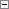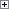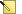C/AL has the following types of variables:

• User-defined variables
• System-defined variables

User-defined variables are variables that you define when you create new C/AL code. You can define variables that are global and apply to all functions in an object, such as a codeunit, or you can define variables that are local and apply to a single function in an object. Both types of user-defined variables are local to the object in which they are defined. These variables can be used to store information at run time, and the values can be changed as desired.

System-defined variables are provided by Microsoft Dynamics NAV. These variables are automatically maintained by the system. Some system-defined variables are Rec, xRec, CurrPage, and CurrReport.

At run time, code is executed in functions and triggers, such as in entry-processing code for a table. Before the code is executed, values are automatically assigned to the associated system-defined variables, and the values of these variables can be used in triggers and local functions.

The values of system-defined variables are not updated when the C/AL code is being executed. Instead, the values are updated before the function or trigger is executed.Note
The value in a system-defined variable does not propagate backward. A user cannot use a system-defined variable to modify the state of the system.

Variable Names

You must follow the following rules and restrictions when you name variables:

• Variable names must be unique. A codeunit cannot contain two user-defined variables with the same name in the same scope. You can have a local variable and a global variable with the same name, but we do not recommend this.
• Uppercase and lowercase letters are not distinct. For example, Smith and SMITH refer to the same variable.
• In C/AL, you can use special characters such as spaces in the name of a variable (an identifier).
• The maximum length of a variable name is 128 characters.
• A variable cannot have the same name as a C/AL function or a reserved word. This applies to both uppercase and lowercase spellings. For example, you cannot use BEGIN or begin as a valid variable name.

All characters in your current ANSI character set are valid in variable names except for the following characters:

• Control characters (ANSI 0-31, 255)
• The quotation mark character (") (ANSI 34)Note
We do not recommend that you use characters that are outside the ASCII characters set, 0-127, because they may display differently on different computers.Note
Microsoft Dynamics NAV runs on the .NET Framework. Therefore, we recommend that you use variables names that are Common Language Specification (CLS)-compliant. For more information, see Common Language Specification in the MSDN Library.

When you name a variable, you cannot use special characters unless you enclose the variable name in quotation marks, as in "Customer No.". If you do not use double quotation marks, then the following rules apply:

• The first character must be one of the following:
• A letter in the range a…z, A…Z (ASCII 97-122, 65-90)
• An underscore (ASCII 95)
• The first character is followed by a maximum of 29 characters, which can be any of the following:
• A letter in the range a…z, A…Z (ASCII 97-122, 65-90)
• An underscore (ASCII 95)
• A digits in the range 0…9 (ASCII 48-57)

You can include one or more special characters in a variable name in C/AL. If you include special characters, then the variable name must be enclosed in quotation marks. In this case, the name can contain any mix of letters, digits, and special characters.Note
The quotation marks are not part of the variable name but are required to successfully compile the codeunit.

Examples

The following examples show valid variable names:

• Customer
• StockGroup1
• "@Vendor"
• "Purchase/Sales"
• "Sales in GBP"
• "Name with Special Characters1Ñ3"

The following examples show incorrect variable names:

• 34467
• 23"Tubes
• Stock Group4
• "Sale"s in GBP"
• )-Names
• END

Initialization

Variables are automatically initialized before C/AL code is executed. A Boolean variable is set to false. Numeric variables are set to the default value zero. Strings (text and code) are initialized to the value '' (an empty string). Date and time variables are set to the undefined time 0T and the undefined date 0D, respectively. Variables of complex data types are also initialized. If a complex data type has multiple components, then each component is initialized to the value that corresponds to the data type for the component.

System-defined variables are automatically handled and initialized. No actions are required by the user before system-defined variables can be used.

Assignment and Type Conversion

You can assign values in the following ways:

• By using the assignment operator ":=", such as Variable := Expression. The data type that results from the evaluation of the right side expression must be the same data type as the variable (left operand) or have a data type that can be converted automatically to the data type of the left operand.
• As parameter assignment, such as FUNCTION(Expression). The data type that results from the evaluation of the expression must correspond to a specific data type or have a data type that can be converted automatically to the correct data type. For more information about evaluation and type conversion in expressions, see Type Conversion in Expressions.

Automatic type conversion in assignments occurs when the following events occur:

• A parameter in a function call does not have the correct data type. For example, this can occur if a function that is supposed to be called by using an integer argument is called by using a DateTime argument.Note
If the value cannot be converted, then a run-time error occurs. If the value can be converted but overflow occurs, then a run-time error occurs.

• The evaluation of the expression on the right side of an assignment operator (:=) achieves a data type that differs from the data type of the variable on the left side, and the expression can be converted to the data type of the variable.

Automatic type conversion in assignments can occur between the following numeric data types as long as an overflow does not occur:

• Byte
• Char
• Integer
• Decimal

Automatic type conversion in assignments can occur between the string data types:

• Code
• Text

Text can be automatically converted to BigText, but a BigText variable must be split into smaller parts before it can be converted to text.

The same assignment rules apply for arrays in C/AL. Furthermore, if the left operand in an assignment (the variable) is an array, the dimension or dimensions of the right side expression must correspond to the dimension or dimensions of the variable.Note
The type conversion that occurs in assignments can cause run-time errors even though the data types are convertible. A run-time error can occur in an assignment if the converted value is outside the valid range for the left side variable. Also, a run-time error can occur if the converted value is outside the valid range for a parameter in a function call.

Example 1

The MyVar variable is defined as a one-dimensional array with four text type elements that have the maximum length 15. This example shows that a value can be assigned to the second element in the array.Copy Code
MyVar:= 'Enter your name';

Example 2

In the following example, the Result variable is an Option variable. The Amount and Total variables are both Decimal variables.Copy Code
Amount := 10;
Total := 4;
...
Result := Amount + Total;

This code can always be compiled, but a run-time error occurs if the result of the right-side expression "Amount + Total" exceeds the range that is permitted by the data type of the left-side Result variable. For an Option variable, the range is the same as the range of an Integer, which is from -2,147,483,647 to 2,147,483,647. A run-time error also occurs if the result of the right-side expression "Amount + Total" is not an integer.

Valid Assignments

The following tables show whether you can assign the value of an expression of a given type to a variable of the same type or to a variable of a different type.

This table shows the numeric data types.

Char expressionOption expressionInteger expressionBigInteger expressionDuration expressionDecimal expression

Char variable

Valid

Valid but overflow may occur

Valid but overflow may occur

Valid but overflow may occur

Valid but overflow may occur

Valid but overflow may occur

Option variable

Valid

Valid

Valid except in a FOR statement

Valid but overflow may occur

Valid but overflow may occur

Valid but overflow may occur

Integer

Valid

Valid

Valid

Valid but overflow may occur

Valid but overflow may occur

Valid but overflow may occur

BigInteger variable

Valid

Valid

Valid

Valid

Valid

Valid but overflow may occur

Duration variable

Valid

Valid

Valid

Valid

Valid

Valid but overflow may occur

Decimal variable

Valid

Valid

Valid

Valid but overflow may occur

Valid but overflow may occur

Valid

This table shows the string data types.

Text expressionCode expressionBigText expression

Text variable

Valid but overflow may occur

Valid but overflow may occur

Valid but overflow may occur

Code variable

Valid but overflow may occur

Valid but overflow may occur

Valid but overflow may occur

BigText variable

Valid but overflow may occur

Valid but overflow may occur

Valid but overflow may occurNote
You can assign the BigText variable with the BigText functions. For more information, see BigText Data Type.